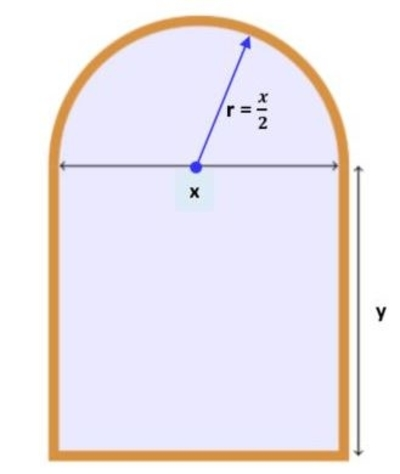# A Norman window has the shape of a rectangle surmounted by a semicircle as in the figure below....

## Question:

A Norman window has the shape of a rectangle surmounted by a semicircle as in the figure below. If the perimeter of the window is 37 ft, express the area, A, as a function of the width, x, of the window.

## Norman Window:

Note that the perimeter is the sum of the sides of the figure and for the norman window its perimeter formula is {eq}\displaystyle P=w+2l+\pi r {/eq} where {eq}w,l,r {/eq} are the width and length of the rectangular part of the widow and {eq}r {/eq} is the radius of the surmounted semicircle. The total area of the window is the sum of the area of rectangle and the area of semicircle.

Below is the figure,Note that the radius of semicircle is one-half of the width of the rectangle. The perimieter is,

{eq}\displaystyle...

Become a Study.com member to unlock this answer! Create your account